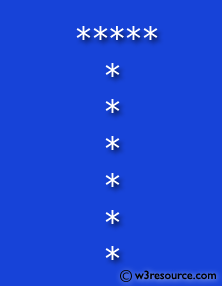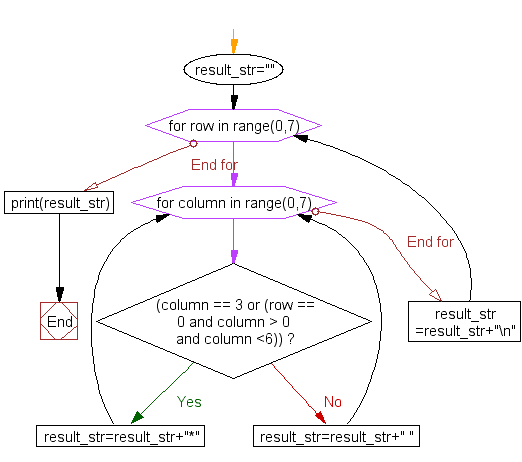﻿ Python Exercise: Print alphabet pattern T - w3resource

# Python Exercise: Print alphabet pattern T

## Python Conditional: Exercise - 27 with Solution

Write a Python program to print the alphabet pattern 'T'.

Pictorial Presentation:Sample Solution:

Python Code:

``````result_str="";
for row in range(0,7):
for column in range(0,7):
if (column == 3 or (row == 0 and column > 0 and column <6)):
result_str=result_str+"*"
else:
result_str=result_str+" "
result_str=result_str+"\n"
print(result_str);
```
```

Sample Output:

``` *****
*
*
*
*
*
*  ```

Flowchart :Python Code Editor:

Have another way to solve this solution? Contribute your code (and comments) through Disqus.

What is the difficulty level of this exercise?

Test your Programming skills with w3resource's quiz.

﻿